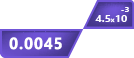# Rational or Irrational Calculator

## Other Calculators

Rational and irrational numbers calculator is a handy tool to detect the type of a number. It is designed to tell you whether the inputted number is rational or irrational.

You can enter numbers in two forms: fraction and decimal since only these types are confusing. It solves the value into decimal form, this means it can also be used as a rational expression/equation calculator.

## How to use this rational or irrational calculator?

To use rational expression calculator follow the below steps.

• Choose the operator i.e division or square root.
• Enter the required values.
• Click "calculate".
• Click the "reset" button to calculate another value.

## What are rational and irrational numbers?

Rational and irrational are the basic types of mathematical numbers. Both of these are defined below.

### Rational:

“Such numbers which you can express in the form of a fraction where the denominator is not zero”

For example 3, 1/9, √4 e.t.c. All of these values are rational because;

• 3 can be written as 3/1.
• 1/9 is already in p/q (fraction) form.
• √4 can be written in the form of a fraction.

All integers, perfect squares, and whole numbers are rational. A property of rational numbers is that either they are finite (stops like 2.457) or infinite and repeating (1.333… ).

### Irrational:

It is the opposite of rational numbers.

“Such numbers which you cannot express in the form of a fraction”

For instance the famous number pi (π) and Euler’s number e. Irrational numbers are infinite and non-repeating.

You can use the rational and irrational number checker to identify any number.

## How to identify rational and irrational numbers?

There are a few tricks that can help to identify the nature of a number manually.

1. Solve the number for decimal form. You can use the equation solver for this purpose.
2. See if the number is finite or infinite. All finite decimal numbers are rational.
3. If it is infinite, see if any of the values are repeating periodically. If yes then the number is rational otherwise irrational.

Example:

Identify the following numbers as rational or irrational. Also, explain the reason.

1. 3.333…
2. ¼
3. 2.6457513110645907
4. √25

#### Identifying:

First number: Rational

Reason: It is infinite recurring (repeating).

Second number: Rational

Reason: It is in p/q form.

Third number: Irrational

Reason: It is infinite but non-recurring.

Last number: Rational

Reason: It is a perfect root.